# NCERT Solutions for Class 8 Maths Exercise 6.3

In this page we have NCERT Solutions for Class 8 Maths Exercise 6.3 .This exercise contains question about how to find the square root using Prime factorization method. Hope you like them and do not forget to like , social share and comment at the end of the page.

## NCERT Solutions for Class 8 Maths Exercise 6.3

Question 1
What could be the possible ‘one’s’ digits of the square root of each of the following numbers?
(i) 9801
(ii) 99856
(iii)998001
(iv)657666025
(i)1 and 9.
Explanation: Since 12 and 92 give 1 at unit’s place, so these are the possible values of unit digit of the square root.
(ii) 4 and 6
Explanation: Since, 42 = 16 and 62 = 36, hence, 4 and 6 are possible digits
(iii) 1 and 9
(iv) 5
Explanation: Since, 52 = 25, hence 5 is possible.

Question 2
Without doing any calculation, find the numbers which are surely not perfect squares.
1. 153
2. 257
3. 408
4. 441
The square of any numbers end in with following digits at its unit place
0,1,4,5,6 or 9
Since (i),(ii),(iii) does not these at its unit place, it can be perfect square of any number, iv) has 1 at its unit place, so it can be square and it is the perfect square of 21

Question 3
Find the square roots of 100 and 169 by the method of repeated subtraction.
(i) Square root of 100 by Repeated subtraction:
1. 100 - 1 = 99
2. 99 - 3 = 96
3. 96 - 5 = 91
4. 91 -7 = 84
5. 84 - 9 = 75
6. 75 - 11 = 64
7. 64 - 13 = 51
8. 51 - 15 = 36
9. 36 - 17 = 19
10. 19 - 19 = 0
We get 0 at 10th step. Thus, 10 is the square root of 100.
(ii) Square root of 169 by Repeated subtraction:
1. 169 - 1 = 168
2. 168 - 3 = 165
3. 165 - 5 = 160
4. 160 - 7 = 153
5. 153 - 9 = 144
6. 144 - 11 = 133
7. 133 - 13 = 120
8. 120 - 15 = 105
9. 105 - 17 = 88
10. 88 - 19 = 69
11. 69 - 21 = 48
12. 48 - 23 = 25
13. 25 - 25 = 0
We get 0 at 13th step. Thus 13 is the square root of 169

Question 4
Find the square roots of the following numbers by the Prime Factorization Method.
1. 729
2. 400
3. 1764
4. 4096
5. 7744
6. 9604
7. 5929
8. 9216
9. 529
(x) 8100
(i) Let find the factor by division of number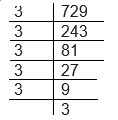So 729 = 32 ×32×32
√729= 27
(ii) Let find the factor by division of number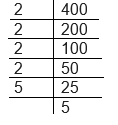So 400 = 22 ×22×52
√400= 20
(iii) Let find the factor by division of number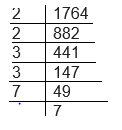So 1764 = 22 ×32×72
√1764= 42
(iv) Let find the factor by division of number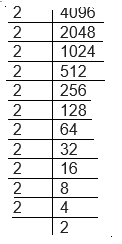So 4096= 22 ×22×22 ×22×22×22
√4096= 64
(v) Let find the factor by division of number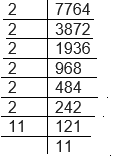So 7744= 22 ×22×22 ×112
√7744= 88
(vi) Let find the factor by division of number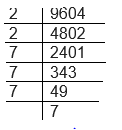So 9604= 22 ×72×72
√9604= 98
(vii) Let find the factor by division of number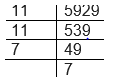So 5929= 112 ×72
√5929= 77
(viii) So 9216= 22 ×22×22×22×22×32
√9216= 96
(ix) So 529= 232
√529= 23
(x) 8100= 22 ×52×32×32
√8100= 90

Question 5
For each of the following numbers, find the smallest whole number by which it should be multiplied so as to get a perfect square number. Also find the square root of the square number so obtained.
(i) 252
(ii) 180
(iii) 1008
(iv) 2028
(v) 1458
(vi) 768
(i) By prime factorization we get,
252 = 2 x 2 x 3 x 3 x 7
Here, 2 and 3 are in pairs but 7 needs a pair. Thus, 7 can become pair after multiplying 252 with 7.
So, 252 will become a perfect square when multiplied by 7.
(ii)
By prime factorization, we get, 180 = 3 x 3 x 2 x 2 x 5
Here, 3 and 2 are in pair but 5 needs a pair to make 180 a perfect square.
180 needs to be multiplied by 5 to become a perfect square.
(iii) By prime factorization of 1008, we get
1008 = 2 x 2 x 2 x 2 x 3 x 3 x 7
Here, 2 and 3 are in pair, but 7 needs a pair to make 1008 a perfect square.
Thus, 1008 needs to be multiplied by 7 to become a perfect square
(iv) By prime factorization of 2028, we get
2028 = 2 x 2 x 3 x 13 x 13
Here, 2 and 13 are in pair, but 3 needs a pair to make 2028 a perfect square.
Thus, 2028 needs to be multiplied by 3 to become a perfect square.
(v) By prime factorization of 1458, we get
1458 = 2 x 3 x 3 x 3 x 3 x 3 x 3
Here, 3 are in pair, but 2 needs a pair to make 1458 a perfect square.
So, 1458 needs to be multiplied by 2 to become a perfect square.
(vi) By prime factorization of 768, we get
768 = 2 x 2 x 2 x 2 x 2 x 2 x 2 x 2 x 3
Here, 2 are in pair, but 3 needs a pair to make 768 a perfect square.
So, 768 needs to be multiplied by 3 to become a perfect square.

Question: 6.
For each of the following numbers, find the smallest whole number by which it should be divided so as to get a perfect square. Also find the square root of the square number so obtained.
(i) 252
(ii) 2925
(iii) 396
(iv) 2645
(v) 2800
(vi) 1620
(i) By prime factorization of 768, we get
252 = 2 x 2 x 3 x 3 x 7
Here, 2 and 3 are in pair, but 7 has no pair, which can be eliminated after dividing 768 by 7.
Hence, 252 needs to be divided by 7 to become a perfect square
(ii) By prime factorization of 2925, we get
2925 = 5 x 5 x 3 x 3 x 13
Here, 5 and 3 are in pair, but 7 has no pair, which can be eliminated after dividing 2925 by 13.
Thus, 2925 needs to be divided by 13 to become a perfect square
(iii) By prime factorization of 396, we get
396 = 2 x 2 x 3 x 3 x 11
Here, 2 and 3 are in pair, but 11 needs another 11 to make a pair. Thus, 11 can be eliminated after dividing 396 by 11.
Thus, 396 needs to be divided by 11 to become a perfect square
(iv) By prime factorization of 2645, we get
2645 = 5 x 23 x 23
Here, 23 is in pair, but 5 needs a pair. Thus, 5 can be eliminated after dividing 2645 by 5
Hence, 2645 needs to be divided by 5 to become a perfect square.
(v) By prime factorization of 2800, we get
2800 = 2 x 2 x 7 x 10 x 10
Since, only 7 is not in pair, thus, by eliminating 7, from 2800, it can be a perfect square.
Hence, 2800 needs to be divided by 7 to become a perfect square.
(vi) By prime factorization of 1620, we get
1620 = 2 x 2 x 3 x 3 x 3 x 3 x 5
Here, 2 and 3 are in pair, but 5 is not in pair. Thus, by eliminating 5 from 1620, obtained number will be a perfect square.
Hence, 1620 needs to be divided by 5 to become a perfect square

Question 7
The students of Class VIII of a school donated Rs 2401 in all, for Prime Minister’s National Relief Fund. Each student donated as many rupees as the number of students in the class. Find the number of students in the class.
We need to calculate the square root of 2401 to get the solution.
By prime factorization of 2401, we get
2401 = 7 x 7 x 7 x 7
√2401= 49
There are 49 students, each contributing 49 rupees

Question 8
2025 plants are to be planted in a garden in such a way that each row contains as many plants as the number of rows. Find the number of rows and the number of plants in each row.
To calculate the number of rows and number of plants, we need to find the square root of 2025.
By prime factorisation of 2025, we get
2025 = 5 x 5 x 3 x 3 x 3 x 3
√2025=45
There are 45 rows with 45 plants in each of them.

Question 9
Find the smallest square number that is divisible by each of the numbers 4, 9 and 10.
In these type of question , we need to  find LCM of 4, 9 and 10
4 = 2 x 2
9 = 3 x 3
10 = 5 x 2
So, LCM = 2 2 x 3 2 x 5 = 180
So 180 is smallest number which is divisible by 4,9,10
But as you see it is not a perfect square
Now the LCM gives us a clue that if 180 is multiplied by 5 then it will become a perfect square.
The Required number = 180 x 5 = 900
Question 10
Find the smallest square number that is divisible by each of the numbers 8, 15 and 20.
8 = 2 x 2 x 2
15 = 3 x 5
20 = 2 x 2 x 5
So, LCM = 2 x 2 x 2 x 3 x 5 = 120
As 3 and 5 are not in pair in LCM’s factor so we need to multiply 120 by 5 and three to make it a perfect square.
Required Number = 180 x 3 x 5 = 2700

## Summary

1. NCERT Solutions for Class 8 Maths Exercise 6.3 has been prepared by Expert with utmost care. If you find any mistake.Please do provide feedback on mail. You can download the solutions as PDF in the below Link also
2. This chapter 6 has total 4 Exercise 6.1 ,6.2,6.3 and 6.4. This is the Third exercise in the chapter.You can explore previous exercise of this chapter by clicking the link below